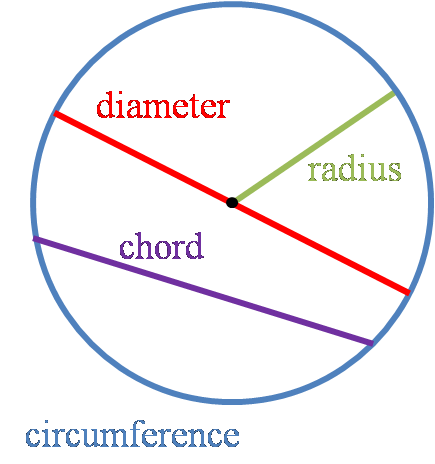# Radius of a Circle Calculator

Created by Maciej Kowalski, PhD candidate
Reviewed by Hanna Pamuła, PhD candidate
Last updated: Sep 25, 2022

Welcome to the radius of a circle calculator, where we'll focus on how to find the radius of a circle from the circumference, area, or diameter. The concept is not too difficult, and, in essence, it's enough to follow a few simple steps we describe in detail below, all of which take you straight to a radius of a circle formula.

## What is the radius of a circle?

By definition, a circle is a 2-dimensional shape consisting of all the points lying at the same, fixed distance from a given point. That distance is known as the radius of the circle.Now that we know what the radius of a circle is (marked with green), let's get familiar with the rest of the lines.

• Circumference (blue) is the perimeter length of the circle.
• The diameter (red) is a line with both endpoints on the circle going through the center.
• Chord (purple) is any line with both endpoints on the circle.

In some sense, the radius is the MVP here: it plays a crucial role in all the formulas, so it's essential to learn how to find the radius of a circle. Fortunately, the task is relatively simple. After all, since the MVP is in all the equations, we can get the circle radius from the area or the radius of a circle from the circumference.

## How do you find the radius of a circle?

There are three circle radius formulas, depending on what number you know:

1. The radius of a circle from the area: if you know the area A, the radius is r = √(A / π).
2. The radius of a circle from circumference: if you know the circumference c, the radius is r = c / (2 * π).
3. The radius of a circle from diameter: if you know the diameter d, the radius is r = d / 2.

Fortunately, our radius of a circle calculator handles all of the above cases. Even better! You don't have to choose which radius of a circle formula you need: simply input the measurement into the tool, and it will automatically process the radius of a circle equation tailored for your needs.

## Other tools like this circle radius calculator

Remember that the radius of a circle calculator is not our only tool dealing with those pesky round objects. Below, we list the others, all ready to deal with your day-to-day circular problems.

## FAQ

### What is the radius of a unit circle?

By definition, a unit circle is a circle with a radius of 1. It is a general object used in mathematics (e.g., when explaining trigonometric functions), so the 1 usually has no unit assigned.

### What is the radius of a 6-foot circle?

To find the radius whose circumference is equal to 6 feet, we follow the steps below:

1. Write the circumference as c = 6 ft.
2. Recall the formula for the radius of a circle from circumference: r = c / (2 * π).
3. Inject the circumference into the equation: r = (6 ft) / (2 * π) = 3/π ft.
4. If needed, substitute π ≈ 3.14: r = 3/π ft ≈ 0.96 ft.
Maciej Kowalski, PhD candidateCircumference (c)
in
Area (A)
in²
Diameter (d)
in
Radius of the circle
in
People also viewed…

### Korean age

If you're wondering what would your age be from a Korean perspective, use this Korean age calculator to find out.

### Multiplying polynomials

The polynomial multiplication calculator is here to find the product of two one-variable polynomials of up to degree 6.

### Right triangle side and angle

How to find the missing side or angle of a right triangle?? We have the answer! Check it with our right triangle side and angle calculator.

### Sleep

The sleep calculator can help you determine when you should go to bed to wake up happy and refreshed.